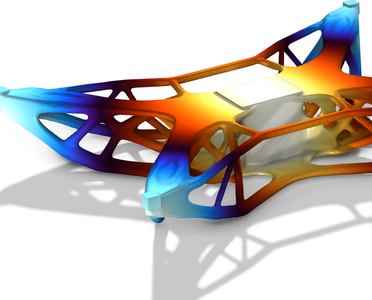# アプリケーションギャラリ

## Topology Optimization of a Drone

Application ID: 98661

Topology optimization for low volume fraction in 3D follows the same methodology as in 2D for medium volume fractions. The only difference is the need for iterative solvers. This model demonstrates topology optimization of a drone for a volume fraction of 12.5 %, while considering two load cases.This model example illustrates applications of this type that would nominally be built using the following products: